## Blur, Noise, and Compression Robust Generative Adversarial Networks

1The University of Tokyo    2RIKEN

CVPR 2021
[Paper] [Slides] [Poster]

We propose blur, noise, and compression robust GAN (BNCR-GAN) that can learn a clean image generator directly from degraded images without knowledge of degradation parameters (e.g., blur kernel types, noise amounts, or quality factor values).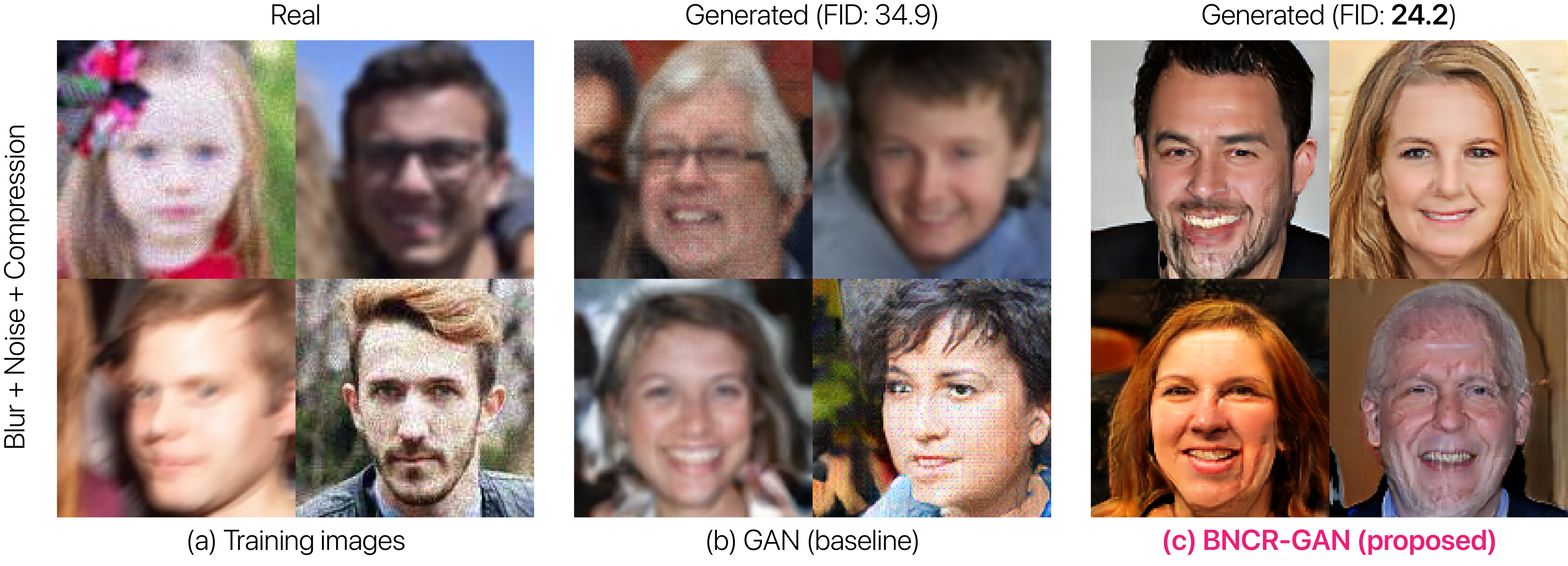Figure 1. Examples of blur, noise, and compression robust image generation. Although recent GANs have shown remarkable results in image reproduction, they can recreate training images faithfully (b), despite degradation by blur, noise, and compression (a). To address this limitation, we propose blur, noise, and compression robust GAN (BNCR-GAN), which can learn to generate clean images (c) even when trained with degraded images (a) and without knowledge of degradation parameters (e.g., blur kernel types, noise amounts, or quality factor values).

#### Our related previous work

 GAN for noise: Noise Robust GAN (CVPR 2020) GAN for label noise: Label-Noise Robust GAN (CVPR 2019) GAN for ambiguous labels: Classifier's Posterior GAN (BMVC 2019)

### Abstract

Generative adversarial networks (GANs) have gained considerable attention owing to their ability to reproduce images. However, they can recreate training images faithfully despite image degradation in the form of blur, noise, and compression, generating similarly degraded images. To solve this problem, the recently proposed noise robust GAN (NR-GAN) provides a partial solution by demonstrating the ability to learn a clean image generator directly from noisy images using a two-generator model comprising image and noise generators. However, its application is limited to noise, which is relatively easy to decompose owing to its additive and reversible characteristics, and its application to irreversible image degradation, in the form of blur, compression, and combination of all, remains a challenge. To address these problems, we propose blur, noise, and compression robust GAN (BNCR-GAN) that can learn a clean image generator directly from degraded images without knowledge of degradation parameters (e.g., blur kernel types, noise amounts, or quality factor values). Inspired by NR-GAN, BNCR-GAN uses a multiple-generator model composed of image, blur-kernel, noise, and quality-factor generators. However, in contrast to NR-GAN, to address irreversible characteristics, we introduce masking architectures adjusting degradation strength values in a data-driven manner using bypasses before and after degradation. Furthermore, to suppress uncertainty caused by the combination of blur, noise, and compression, we introduce adaptive consistency losses imposing consistency between irreversible degradation processes according to the degradation strengths. We demonstrate the effectiveness of BNCR-GAN through large-scale comparative studies on CIFAR-10 and a generality analysis on FFHQ. In addition, we demonstrate the applicability of BNCR-GAN in image restoration.

### Key ideas

To solve the sub-problems, we first propose two variants: blur robust GAN (BR-GAN) and compression robust GAN (CR-GAN), which are specific to blur and compression, respectively. To address the irreversible blur/compression characteristics, masking architectures adapting degradation strengths in a data-driven are introduced, using bypasses before and after image degradation. This architectural constraint is useful for conducting only the necessary changes through blur or compression while suppressing unnecessary changes.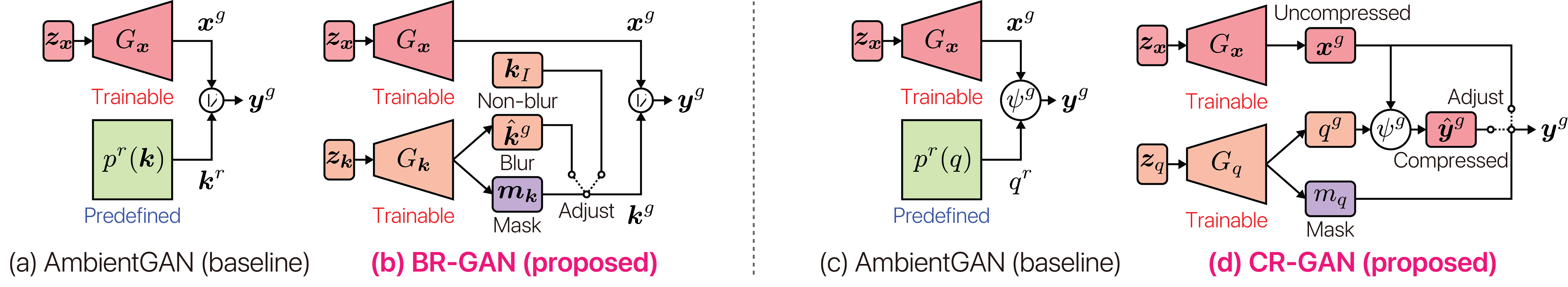Figure 2. Comparison of AmbientGANs (baseline), BR-GAN (proposed), and CR-GAN (proposed). Because the discriminators are the same for all models, we only depict the generators. (a)(c) AmbientGANs assume that blur and compression simulation models (i.e., $$p^r (\boldsymbol{k})$$ and $$p^r (q)$$) are predefined. (b)(d) In order to eliminate this assumption, we introduce a blur-kernel generator $$G_{\boldsymbol{k}}$$ in BR-GAN (b) and a quality-factor generator $$G_q$$ in CR-GAN (d), and train them along with clean image generators $$G_{\boldsymbol{x}}$$. In BR-GAN (b), we introduce a masking architecture adjusting the balance between a generated kernel $$\hat{\boldsymbol{k}}^g$$ (i.e., blur) and an identity kernel $$\boldsymbol{k}_{I}$$ (i.e., non-blur) based on a mask $$\boldsymbol{m}_{\boldsymbol{k}}$$. Similarly, in CR-GAN (d), we incorporate a masking architecture adjusting the balance between a compressed image $$\hat{\boldsymbol{y}}^g$$ and an uncompressed image $$\boldsymbol{x}^g$$ based on a mask $$m_q$$. Note that every parameter (i.e., $$\hat{\boldsymbol{k}}^g$$, $$\boldsymbol{m}_{\boldsymbol{k}}$$, $$q^g$$, and $$m_q$$) is trainable, and is determined in a data-driven manner.

The unique problem of BNCR-GAN, which is a unified model integrating BR-GAN, NR-GAN, and CR-GAN, is that it needs to handle the uncertainty caused by the combination of blur, noise, and compression. Thus, we incorporate novel losses called adaptive consistency losses that impose consistency between irreversible degradation processes according to the degradation strengths. This loss helps prevent the generated image from yielding unexpected artifacts, which can disappear and become unrecognizable after irreversible processes.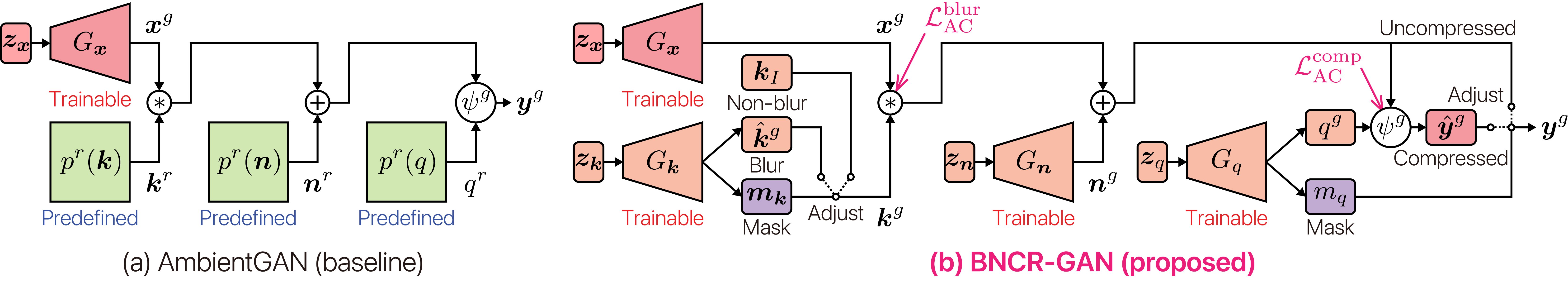Figure 3. Comparison of AmbientGANs (baseline) and BNCR-GAN (proposed). We depict only the generators because the discriminators are the same for both models. (a) AmbientGAN assumes that blur, noise, and compression simulation models (i.e., $$p^r (\boldsymbol{k})$$, $$p^r (\boldsymbol{n})$$, $$p^r (q)$$) are predefined. (b) To reduce this assumption, we introduce a blur-kernel generator $$G_{\boldsymbol{k}}$$, a noise generator $$G_{\boldsymbol{n}}$$, and a quality-factor generator $$G_q$$ and train them along with a clean image generator $$G_{\boldsymbol{x}}$$ with adaptive consistency losses (i.e., $$\mathcal{L}_{\text{AC}}^{\text{blur}}$$ and $$\mathcal{L}_{\text{AC}}^{\text{comp}}$$, which impose consistency between before and after blur and compression according to the blur and compression strengths (i.e., $$\boldsymbol{k}^g$$ and $$q^g$$), respectively.

### Example results

#### Examples of blur robust image generation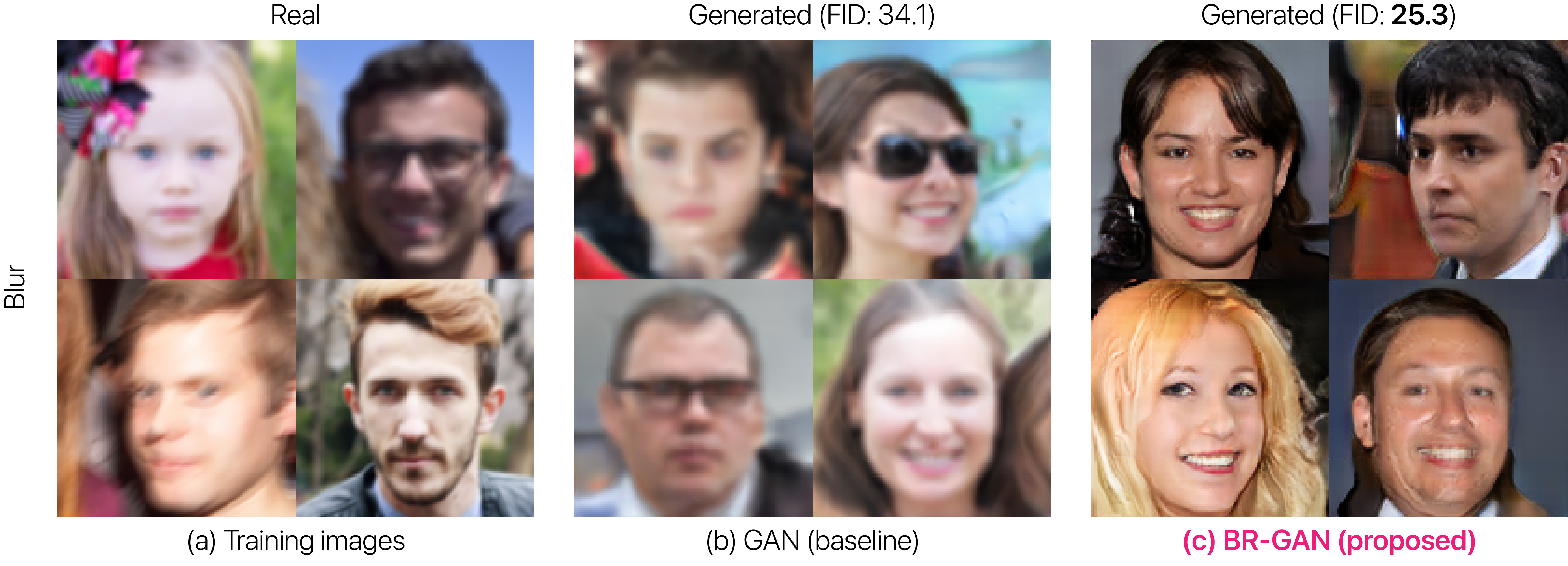Figure 4. Examples of blur robust image generation. Standard GAN can recreate training images faithfully (b) despite degradation by blur (a). In contrast, BR-GAN can learn to generate clean images (c) even when trained with blurred images (a) and without knowledge of blur parameters (i.e., blur kernel types).

#### Examples of compression robust image generation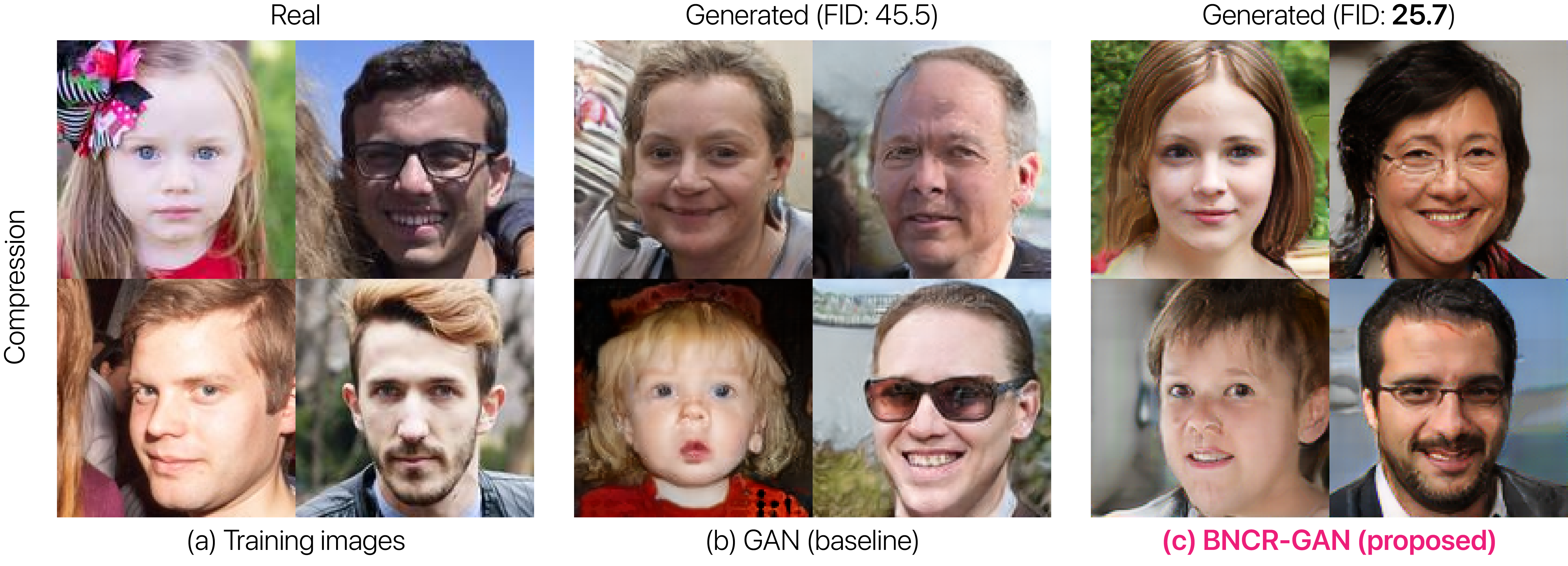Figure 5. Examples of compression robust image generation. Standard GAN can recreate training images faithfully (b) despite degradation by compression (a). In contrast, CR-GAN can learn to generate clean images (c) even when trained with compressed images (a) and without knowledge of compression parameters (i.e., quality factor values).

#### Examples of blur, noise, and compression robust image generationFigure 6. Examples of blur, noise, and compression robust image generation. Standard GAN can recreate training images faithfully (b) despite degradation by blur, noise, and compression (a). In contrast, BNCR-GAN can learn to generate clean images (c) even when trained with blurred, noisy, and compressed images (a) and without knowledge of blur, noise, and compression parameters (e.g., blur kernel types, noise amounts, and quality factor values).

### Paper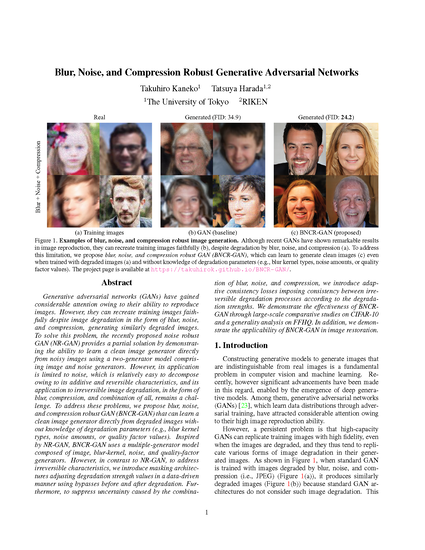Takuhiro Kaneko and Tatsuya Harada. Blur, Noise, and Compression Robust Generative Adversarial Networks. In CVPR, 2021. (arXiv:2003.07849, Mar. 2020) [Paper] [BibTex]

### Acknowledgment

This work was partially supported by JST AIP Acceleration Research Grant Number JPMJCR20U3, JST CREST Grant Number JPMJCR2015, and JSPS KAKENHI Grant Number JP19H01115.

### References

 A. Bora, E. Price, A. G. Dimakis. AmbientGAN: Generative Models from Lossy Measurements. In ICLR, 2018.
 T. Kaneko, T. Harada. Noise Robust Generative Adversarial Networks. In CVPR, 2020.
 T. Kaneko, Y. Ushiku, T. Harada. Label-Noise Robust Generative Adversarial Networks. In CVPR, 2019.
 T. Kaneko, Y. Ushiku, T. Harada. Class-Distinct and Class-Mutual Image Generation with GANs. In BMVC, 2019.